# User:IssaRice/Computability and logic/Some important distinctions and equivalences in introductory mathematical logic

This page lists some important distinctions and equivalences in introductory mathematical logic and computability theory. Bizarrely, most books won't even mention these distinctions, so you will probably be very confused at the start as you inevitably conflate the distinct ideas and can't confidently make the connection between equivalent ideas.

This page is kind of a "list of things I wish someone told me" as I was learning basic logic. It's not intended as a standalone introduction to mathematical logic, but rather a supplement to more standard resources. I imagine this page being too easy or completely obvious to people who have studied mathematical logic, and incomprehensible to people who have not studied it. There should be a "sweet spot" somewhere in between, of someone who is struggling with making sense of mathematical logic. In particular, if you are working through Boolos/Burgess/Jeffrey, this might be the post for you!

For the LessWrong audience: (1) there have been a number of posts on mathematical logic before; (2) some folks are studying this material in preparation for doing AI alignment research; (3) making distinctions between concepts that seem similar at first seems like a useful rationality skill  . Other applications? (metaethics, buckets errors, "your strength as a rationalist" vs "your consistency as a theory")

## Completeness

The term "complete" can apply to a logic, in which case it is also called "semantically complete". If a logic is semantically complete, it means that if a set of sentences$\Delta$ semantically implies a sentence$\phi$ (i.e. every interpretation that makes every sentence in$\Delta$ true also makes$\phi$ true), then it is possible to prove$\phi$ using$\Delta$ as the set of assumptions. This meaning of completeness is the topic of Gödel's completeness theorem. In addition, this form of completeness can be stated in another way by saying that any consistent set of sentences is satisfiable (has a model); some proofs of Gödel's completeness theorem use this formulation (for a proof of the equivalence, see here).

The term "complete" can also apply to a theory, in which case it is also called "negation-complete". If a theory is negation-complete, it means that for every sentence$\phi$, the theory proves either$\phi$ or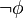$\neg \phi$ (if it proves both, it is still negation-complete, but it is also inconsistent, so is not an interesting theory). This meaning of completeness is the topic of Gödel's first incompleteness theorem (which states that certain theories of interest are not negation-complete). Completeness for theories can also be stated in the following way: a theory$T$ is incomplete iff it does not decide every sentence (i.e. there exists an undecidable sentence).

These two ideas are actually related. See Peter Smith's footnote on this.

The lesson with completeness is to always be sure what kind of object it is being applied to.

outside of logic, in mathematics there are many different meanings of "completeness". However, these will be pretty clearly different, since the context is so different.

Exercise. (Give the one about how goedel's completeness and incompleteness theorems seem contradictory)

## Soundness

soundness: sound logic (soundness theorem) vs sound theory. soundness of logic is about truth in all interpretations, while soundness of theory is about truth in a specific interpretation. unless the axioms of a theory are just valid sentences (in which case, the axioms are not really adding anything beyond what the logic already has, assuming the logic is complete), the axioms of a theory will in general be false in some interpretations -- this is what makes theories interesting, because they have non-logical content.

## Truth in intended interpretation vs truth in all interpretations

Let$P$ be a predicate, and let$c$ be a constant. Is the sentence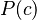$P(c)$ true? You would rightly say that the truth depends on what meanings we gave to the predicate and the constant. What about the sentence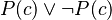$P(c)\vee \neg P(c)$? Now we might be tempted to say that this sentence is true. Someone might say "But you don't even know what$P$ and$c$ mean! How can you know if it's true or not?" One might respond that no matter what meanings we gave to$P$ and$c$, the sentence would come out true. In other words, there is no way to assign meanings to$P$ and$c$ that would make the sentence false.

truth in all interpretations (validity) vs truth in the intended interpretation (natural reading, standard interpretation): see User:IssaRice/Computability and logic/Intended interpretation versus all interpretations

## The models symbol

The models symbol,$\models$, comes up a lot in logic. But its meaning can be quite different depending on how it is used.

The two basic variations are:

• When a set of sentences comes before the symbol, like in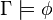$\Gamma \models \phi$. In this case, we are talking about the truth in all interpretations. When$\Gamma = \emptyset$, it is sometimes omitted, so that we just write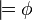$\models \phi$.
• When a structure comes before the symbol, like in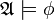$\mathfrak A \models \phi$. In this case, we are talking about the truth in just that one structure.
•$\models$: when a set of sentences comes before the symbol vs when a structure comes before the symbol: see User:IssaRice/Computability and logic/Models symbol

## Syntax vs semantics

• "proves" vs "semantically implies" (absence of counterexample)
• deduction vs truth tables

In computability theory, "syntax" corresponds to algorithms and "semantics" corresponds to functions computable via algorithms. See Rice's theorem, where this distinction becomes especially important.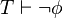$T \vdash \neg \phi$ is very different from$T \not\vdash \phi$, but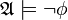$\mathfrak A \models \neg \phi$ is equivalent to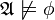$\mathfrak A \not\models \phi$ (in fact, the latter is the definition of the former!).

Exercise. Is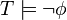$T \models \neg \phi$ is equivalent to$T \not\models \phi$?

## Sentence vs wff vs formula vs closed formula

• formula = wff (you can read some of the history here)
• sentence = closed formula
• the exception is that in propositional logic, since there are not quantifiers, it doesn't makes sense to distinguish between formulas and sentences, so I think everyone just calls these things formulas.

## Enumerating vs computably enumerating vs primitive-recursively enumerating

First of all, there's a lot of terms that mean the same thing, so to clarify: "computably enumerable" means "recursively enumerable" means "effectively enumerable" (although the last one might be used as the informal counterpart, so would be equivalent to the formal counterparts only given the Church-Turing thesis).

Let$A$ be a set of natural numbers. We might say that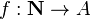$f : \mathbf N \to A$ enumerates$A$ iff$f$ is surjective. Why? because we can list out the elements of$A$ as follows: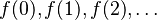$f(0), f(1), f(2), \ldots$. Since$f$ is surjective, every element of$A$ will show up in this list at some point, i.e. we have "enumerated" the elements of$A$. Call a set enumerable if there is a function that enumerates it. For those who have studied countability/uncountability, the definition of "enumerable" given here is the same as "at most countable".

The idea in computability theory is that we don't want just any enumeration. Since we are studying computable functions, we want to restrict our attention to computable enumerations. This leads to the idea of computably enumerable sets.

Given that primitive recursive functions are a strictly smaller class compared to partial recursive functions, one might suspect that sets which can be enumerated by primitive recursive functions are also a smaller class compared to sets which can be enumerated by partial recursive functions. To put it another way, suppose that a set is primitive-recursively enumerable. Then we have some primitive recursive function$f$ that enumerates it. But primitive recursive functions are recursive, so$f$ is recursive. Thus the set is recursively enumerable. That's one way, but it's not obvious whether the converse holds. It turns out that the converse does hold.

NOTE: in Solomonoff induction and related areas there is a concept of "enumerable" which is different from the enumeration used here.

Exercise (from boolos). Show that enumerable = "at most countable".

## algorithm vs program vs source code vs index vs Godel number

I think all of these are essentially the same in that they are all ways of encoding some objects one cares about, but I notice that my "mental imagery" is different for them.

Formulas are identified by their syntax (how they are written) rather than by their meaning, whereas computable partial functions are identified by their meaning (behavior) rather than their syntax. This means that if you are told a partial function is computable you can find some algorithm that computes it, but it won't be "the" algorithm, since there are many algorithms that compute it. In the case of formulas, you can find the formula given a godel number.

• Godel number I think is used most in logic when numbering formulas. It's also the case that when numbering formulas, no two godel numbers have the same formula, i.e. the mapping from godel numbers to formulas is injective. (This is not the case when numbering computable functions.)
• When I think of "algorithm" or "program", I imagine it being a string rather than a natural number (it seems most concrete to me to imagine a Python program). This distinction turns out to not be important, since we can just think of strings as integers. The term "algorithm" especially makes me think about the actual behavior, whereas with "index" I don't think about it.
• When I think of "index" I think of natural numbers. I also imagine there being some sequence of indices, whereas with "algorithm" or "program" I can think of them in isolation.

TODO: mention lambda calculus, where there is no distinction between the different "levels", so e.g. we get a fixed point result without doing any encoding.

## theory vs axioms

I think "theory" and "axioms" can often be used interchangeably in logic. For instance, to say that a theory proves a sentence is the same as saying the axioms prove the sentence, since everything in the theory comes from the axioms.

Synecdoche problem: in mathematics more generally, when referring to a structured space (such as a group) it is conventional to use the same name for both the space itself as well as the set of objects living in that space.See these two comments by Terence Tao. I think a similar thing happens with the word "theory" in logic. Some books say a theory is just a set of sentences -- any set of sentences. Other books require that this set be closed under deductions (it would be pretty strange to have a theory where$p$ and$q$ are theorems but something deducible from them, such as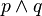$p\wedge q$, is not a theorem). Yet other books single out the starting set of sentences (as non-logical axioms) and then call the set of sentences that can be proved from it the theorems; the "theory" is then the overall name applied to the whole structure.

In practice this distinction doesn't seem to matter much.

## Deciding

The terms "decides", "decidable", "deciding", etc. show up a lot in computability and logic. There are several meanings here, which makes things pretty confusing.

• Decidable set or relation: given an input in some class (e.g. a natural number) there must be an algorithm that tells us whether or not the input is in the set or has the property. The thing to contrast "decidable" is with recognizing or semi-deciding, which says that if the input is in the set, we must say it is in a finite amount of time, but if it isn't, then we don't need to produce any answer.
• Decidable theory: given a sentence, we must say whether or not the sentence is provable in the theory. This is an instance of the previous point, if we think of a theory as a set of sentences.
• A theory deciding a sentence: a theory$T$ decides a sentence$\phi$ if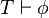$T \vdash \phi$ or$T \vdash \neg\phi$. In other words, the theory must prove or refute the sentence. Note that saying$T \vdash \neg\phi$ is very different from saying$T \not\vdash \phi$. This means that a theory is negation-complete iff it decides every sentence.
• Decidable logic: to say that a logic (such as propositional logic or first-order logic) is decidable means that there is an algorithm that will tell us whether or not any given sentence is valid (i.e. true under every interpretation). In the case of first-order logic, the question of whether it is decidable is called the Entscheidungsproblem. Note also that the decidability of first-order logic can be stated in several equivalent ways.

## algorithm vs function computed by algorithm

Things that algorithms have that functions computable by algorithms don't have:

• The type$\mathbf N$
• A runtime
• A specific memory usage (note: you can prove things about functions like "it can't be computed using less than such-and-such memory", but you can always use more memory to compute a function, so there isn't a specific number)

Things that functions computable by algorithms have that algorithms don't have:

• The type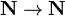$\mathbf N \to \mathbf N$

### Diagonalization redpill

As you've gone along studying computability and logic, I'm sure you've had thoughts like "hmm, Cantor's theorem, Russell's paradox, and the halting problem all seem pretty much the same" or "the recursion theorem and the diagonalization lemma seem suspiciously similar". You may even have heard of Lawvere's theorem, which is supposed to tie all these things together, but you may have said "eh, I don't know any category theory and I'm not sure it's worth learning it just to understand diagonalization better".

My suggestion is to eventually read Yanofsky's paper, "A Universal Approach to Self-Referential Paradoxes, Incompleteness and Fixed Points". The paper covers Lawvere's theorem and ties all the diagonalization results together without using any category theory. The paper is highly readable (assuming you've already studied computability and logic). I suggested reading the paper "eventually" because I'm not sure reading it immediately before/after studying logic produces the best "emotional impact". Personally, I found the paper after struggling a lot with trying to make sense of diagonalization (specifically, I had been spending a few days trying to see how the recursion theorem and diagonalization lemma were the same).

Exercise. Find the flaw in yanofsky's recursion theorem proof.

### Reverse mathematics

Stillwell's book.

## Language, logic, formal system, system, theory, structure

Nate Soares already covered some of this in his post.

Here's a table that might help to keep things straight.

Name Examples
Language
Signature
Logic Propositional logic, first-order logic
Theory Robinson arithmetic, Peano arithmetic
Structure The natural numbers
Proof system Hilbert system, natural deduction

language/signature manage the symbols

logic (and arity function of signature) manage the syntax

theory/axioms manage the content-specific rules of how to push symbols around

proof system manages the content-agnostic rules of how to push symbols around

structure manages how to interpret the symbol pushing